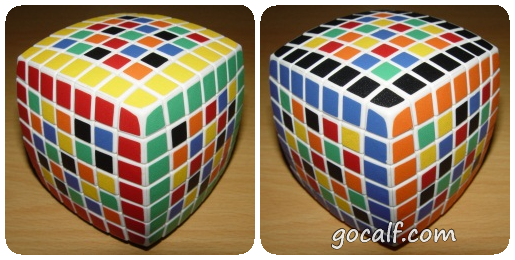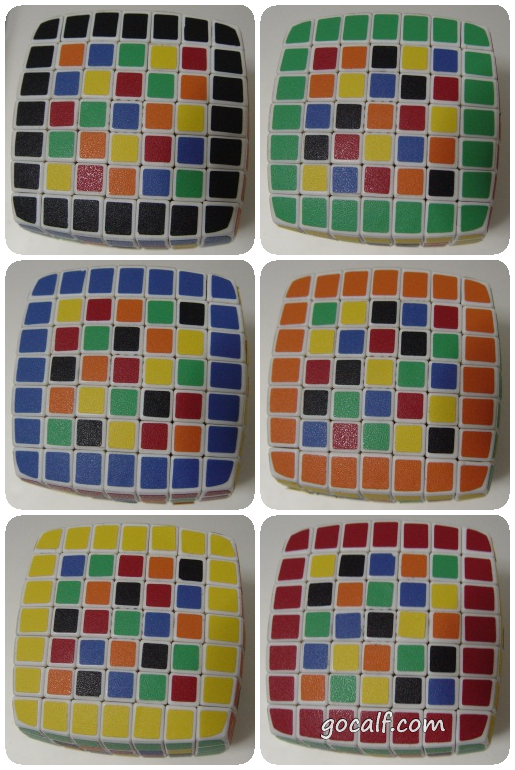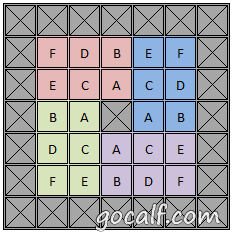``` 1 2 3 4 5 6 7 8 9 10 11 12 13 14 15 16 17 18 19 20 21 22 23 24 25 26 27 28 29 30 31 32``` ```def FillBoard(board, n, xy): x = xy % n y = xy // n if y >= n: print('Find a possible solution.') return True existValues = set() existValues = existValues.union([board[y][xx] for xx in range(n)]) existValues = existValues.union([board[yy][x] for yy in range(n)]) if x == y: existValues = existValues.union([board[xx][xx] for xx in range(n)]) if x + y == n - 1: existValues = existValues.union([board[xx][n-xx-1] for xx in range(n)]) validValues = [v for v in range(1, n+1) if v not in existValues] if not validValues: return False for v in validValues: board[y][x] = v if FillBoard(board, n, xy+1): return True board[y][x] = None return False def GenerateBoard(n): board = [[None for x in range(n)] for y in range(n)] if not FillBoard(board, n, 0): print('No solution.') return board cnt = 5 board = GenerateBoard(cnt) ```

 ```1 2 3 4 5``` ```[1, 2, 3, 4, 5] [2, 4, 5, 3, 1] [5, 3, 2, 1, 4] [3, 1, 4, 5, 2] [4, 5, 1, 2, 3] ```

 ``` 1 2 3 4 5 6 7 8 9 10 11 12 13 14 15 16 17 18 19 20 21``` ```def ApplyColor(board, colors): newboard = [[colors[v] for v in row] for row in board] return newboard def DrawBoard(board): for row in board: print(row) allcolors = ( ['B Center', 'R', 'B', 'O', 'W', 'Y'], ['R Center', 'G', 'R', 'B', 'W', 'Y'], ['G Center', 'O', 'G', 'R', 'W', 'Y'], ['O Center', 'G', 'O', 'B', 'W', 'Y'], ['Y Center', 'R', 'Y', 'O', 'B', 'G'], ['W Center', 'R', 'W', 'O', 'G', 'B'], ) for colors in allcolors: print('Colors:', colors) colorboard = ApplyColor(board, colors) DrawBoard(colorboard) print() ```

So what do you think? Did I miss something? Is any part unclear? Leave your comments below.